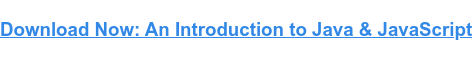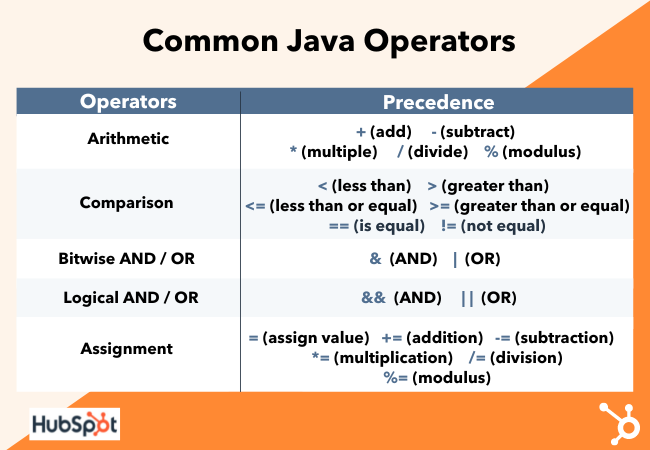# What Does += Mean in Java?Updated:

Published:

When first learning to code, all the symbols and operators can be daunting. But as you get a feel for the language, you begin to understand what they all mean. The += operator in Java is one of those operators that can stump new programmers.If you're new to the world of Java programming or just need a refresher, you may be wondering what the += operator means. This operator is known as the addition assignment operator, and it is a very handy operator to know.

This blog post will explain what it does and how to use it. Let's get started.

## What are Java operators?

Operators are symbols that perform specific operations on one or more operands. In Java, there are a few different types of operators that you can use. The most common ones are the arithmetic operators, which include the addition, subtraction, multiplication, and division operators. There are also assignment operators, which include the = operator that you use to assign values to variables. And then, there are the comparison operators, which allow you to compare two values and see if they are equal, greater than, or less than each other.In this case,  we are interested in the addition assignment operator, which is represented by the += symbol. Let's go into more detail.

## What does += Operator mean in Java?

The addition assignment operator, +=, is a shorthand way to add a value to a variable. The code x+=y is equivalent to x=x+y. This can be used with any primitive data type: char, byte, short, int, long, and float. You can also use it with Strings, though technically, this is not an addition but a concatenation.

To put it simply, the addition assignment operator is a combination of the addition and assignment operators. So what does that mean? Let's say you have two variables, x, and y, and you want to add their values together and then store the result in a third variable, z. You could do this by using the following code:

x = x + y; z = x;

This would add the values of x and y together and then store the result in z. But what if you wanted to do this in one line of code? That's where the addition assignment operator comes in. You can use it like this:

z += x + y;

This will add the values of x and y together and then store the result in z. The addition assignment operator is a shorthand way to write this code, and it can be convenient when you're working with large amounts of data.

So what's the difference between this and the code above? Well, using the addition assignment operator is a lot simpler and more concise. Plus, it can help you avoid mistakes when adding two values together.

## How to Use the Java += Operator

Now that you know what the addition assignment operator does, let's look at how to use it. As we mentioned, the addition assignment operator adds two values together and then assigns the result to a variable. Let's say you have a variable x with the value of 100, and you want to add 20 to it. You could do this by using the following code:

x += 20;

This would add 20 to the value of x and then store the result in x. So now, if you print out the value of x, it would be 120.

You can also use the addition assignment operator to concatenate strings. For example, let's say you have a string called str with the value "Hello." If you wanted to add the string "World" to it, you could do this:

str += "World";

This would concatenate the two strings and then store the result in str. So now, if you were to print out the value of str, it would be "Hello World".

As you can see, the addition assignment operator is a convenient tool to know. It can help you add two values together quickly and easily, and it can also help you avoid making mistakes when adding numbers or concatenating strings.

## Examples of += in Java

Let's look at a few examples of the addition assignment operator in action.

In this example, we're going to add two numbers together and store the result in a variable.

int x = 100; int y = 20; int z = 0; z += x + y; System.out.println("z is: " + z); // 120

In this code, we've declared three variables, x, y, and z. We've assigned values to x and y and then added them together using the addition operator (+). The result of this operation is stored in the z variable. We've then printed out the value of z to the console to see what it is.

### Example #2: Concatenating Strings

In this example, we will combine two strings and then store the result in a variable.

String str = "Hello"; str += " World"; System.out.println(str); // Hello World

In this code,  we've declared a string variable called str and assigned it the value "Hello". We've then concatenated the string " World" to it using the addition assignment operator. The result of this operation is stored in the str variable. We've then printed out the value of str to the console to see what it is.

### Example #3: Adding Numbers and Concatenating Strings

In this example, we will add two numbers together and store the result in a variable. We'll also use the += operator to concatenate a string to our variable.

int x = 100; int y = 20; String str = "z is: ";  str += x + y; System.out.println(str); // z is: 120

In this code,  we've declared two variables, x and y. We've assigned values to them and then added them together using the addition operator (+). The result of this operation is stored in a string variable called str. We've concatenated the string "z is: " to our variable using the addition assignment operator, and then we've printed out the value of str to the console.

### Example #4: Java Loop with += Operator

In this example, we will use the addition assignment operator in a loop. We'll add the numbers 0 through 100 together and then store the result in a variable called sum.

int sum = 0; for(int i=0; i <= 100; i++) { sum += i; } System.out.println("The sum is: " + sum); // The sum is: 5050.

In this code, we've declared a variable called sum and assigned it the value 0. We've then created a for loop that loops 100 times.

Each time the loop runs, we add the value of i to our sum variable. When the loop has finished running, we're printing out the value of sum to the console.

## When should you use += in Java?

In addition to using the addition assignment operator for basic math operations, you can also use it to concatenate strings together. This makes the addition assignment a very versatile tool that can come in handy in various situations. So, if you're looking for a quick and easy way to add two values together or concatenate strings, the addition assignment operator is the tool for you.

Now that you know what the addition assignment operator is and how to use it put it to good use in your own Java programs.Topics: Java

## Related Articles

We're committed to your privacy. HubSpot uses the information you provide to us to contact you about our relevant content, products, and services. You may unsubscribe from these communications at any time. For more information, check out our Privacy Policy.